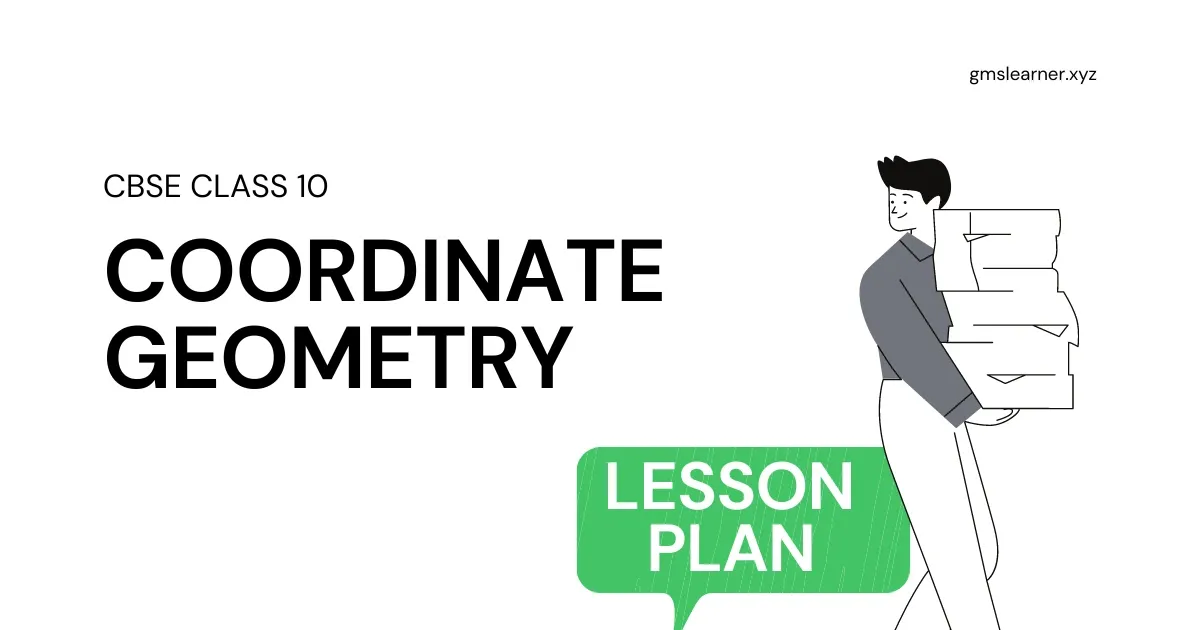Coordinate Geometry Lesson Plan &amp; Activities For Class 10 | Materials, Curriculum Standards, Key Vocabulary, Instructions &amp; more - Padhle.Online
Students' favourite free learning app with LIVE online classes, instant doubt resolution, unlimited practice for classes 6-12, personalized study app for Maths, Science, Social Studies, video e-learning, online tutorial, and more. Download Now!

# Coordinate Geometry Lesson Plan & Activities For Class 10 | Materials, Curriculum Standards, Key Vocabulary, Instructions & more

Don't get off track in your instruction on coordinate geometry. A simple and direct text lesson walks students through basic definitions, principles, formulas, equations and their solutions to prepare them for independent work.Coordinate Geometry Lesson Plan & Activities For Class 10 | Materials, Curriculum Standards, Key Vocabulary, Instructions  & more

 Board - CBSE CLASS –X SUBJECT- MATHEMATICS CHAPTER 7 : Coordinate Geometry

TOPIC:-
Chapter 7 : Coordinate Geometry

DURATION:-
This chapter is divided into Eight modules and it is completed in fifteen class meetings.

PRE- REQUISITE KNOWLEDGE:-
Knowledge of coordinate geometry class 9th .
Properties of different types of quadrilaterals chapter 8 class 9th .

TEACHING AIDS:-
Green Board, Chalk,  Duster, Charts, smart board, laptop, projector  etc.

METHODOLOGY:-
Demonstration and Lecture method.

OBJECTIVES:-
• Concept and introduction of coordinate geometry.
• Graphs of linear equations and method of representing the ordered pairs on the graph.
• Distance formula  and its applications in different problems.
• Section formula (internal division of the line), mid- point formula and the related problems.
•  Area of triangle and method of proving that three points are collinear.

PROCEDURE :-
Start the session by checking their previous knowledge, by asking the questions related to the Cartesian coordinate system and the method of representing them on  the graphs. After this explain the topic to the students step by step as follows.

 S No TOPIC[For Complete Explanation of the topic] 1 Introduction of coordinate geometryCoordinate geometry is the combination of Algebra and Geometry. Before start this topic teacher will explain the Cartesian coordinate system. Here teacher will explain Horizontal line, Vertical line, ordinate, abscissa, point on x-axis, point on y-axis, origin, quadrant etc. 2 Distance between two pointsPlot two points on the graph and then derive the distance formula by applying the Pythagoras theorem. Distance between two points A(x1,y1) and B(x2, y2) is given byd=|AB|=(x2−x1)2+(y2−y1)2−−−−−−−−−−−−−−−−−−√$d=|AB|=\sqrt{\left({x}_{2}-{x}_{1}{\right)}^{2}+\left({y}_{2}-{y}_{1}{\right)}^{2}}$ 3 Collinearity of three points by distance formulaExplain the concept of collinearity of three points by using the distance formula.Three or more points are said to be collinear if all the points lie on the straight line.In order to prove that three points are collinear we shall prove the following statement.If A(x1, y1) , B(x2, y2) and C(x3, y3) are three points on the line then|AB| + |BC| = |AC| 4 Conditions for different types of quadrilateralWith the help of distance formula we can find the types of quadrilateral by using the following concepts.Parallelograms: Opposite sides are equal but diagonals are not equal.Rectangle: Opposite sides are equal and diagonals  are also  equal.Rhombus: All sides are equal but diagonals are not equal.Square: All sides are equal and diagonals are also equal.Teacher will explain the concept by taking some examples. 5 Now teacher will Explain the problems related to the following statements.Any point on the x-axis is (a, 0).Any point of the y-axis is (0, b)Any point given equidistant from two given points. 6 Section FormulaNow teacher will Explain the derivation of section formula and its implementation in the different problems.If A(x1,y1) and B(x2, y2) are two points on the straight line  and if point P(x, y) divide the line between A and B in m1 : m2, then by section formula:P(x,y)=(m1x2+m2x1m1+m2,m1y2+m2y1m1+m2)$P\left(x,y\right)=\left(\frac{{m}_{1}{x}_{2}+{m}_{2}{x}_{1}}{{m}_{1}+{m}_{2}},\frac{{m}_{1}{y}_{2}+{m}_{2}{y}_{1}}{{m}_{1}+{m}_{2}}\right)$Teacher will also explain the implementation of this formula in different problems. Teacher may also provide some problems to the students. 7 Mid Point FormulaAfter this teacher will introduce the Mid-Point formula and give some problems to the students for practice.If A(x1,y1) and B(x2, y2) are two points on the straight line  and if point P(x, y) is the mid - point of AB, then by Mid-Point formulaP(x,y)=(x1+x22,y1+y22)$P\left(x,y\right)=\left(\frac{{x}_{1}+{x}_{2}}{2},\frac{{y}_{1}+{y}_{2}}{2}\right)$ 8 Area of TriangleNow teacher will introduce the formula of finding the area of triangle and extend this formula to find the area of the quadrilateral.If A(x1, y1) , B(x2, y2) and C(x3, y3) are three vertices of a triangle then area of triangle is given by=12[x1y2+x2y3+x3y1−(y1x2+y2x3+y3x1)]$=\frac{1}{2}\left[{x}_{1}{y}_{2}+{x}_{2}{y}_{3}+{x}_{3}{y}_{1}-\left({y}_{1}{x}_{2}+{y}_{2}{x}_{3}+{y}_{3}{x}_{1}\right)\right]$Explanation of the formulaThis formula can also be used to find the area of quadrilateral as follows=12[x1y2+x2y3+x3y4+x4y1−(y1x2+y2x3+y3x1+y4x1)]$=\frac{1}{2}\left[{x}_{1}{y}_{2}+{x}_{2}{y}_{3}+{x}_{3}{y}_{4}+{x}_{4}{y}_{1}-\left({y}_{1}{x}_{2}+{y}_{2}{x}_{3}+{y}_{3}{x}_{1}+{y}_{4}{x}_{1}\right)\right]$ 9 Collinearity of three points by using area of triangleThree points are collinear if area of the triangle is equal to zero. Teacher will assign some problems to the students  to apply this concepts. 10 Centroid of TriangleNow teacher will give the knowledge of centroid of the triangle and suggest the formula used to find it. If A(x1, y1) , B(x2, y2) and C(x3, y3) are three vertices of a triangle then Centroid P(x,y) of triangle is given byP(x,y)=(x1+x2+x33,y1+y2+y33)$P\left(x,y\right)=\left(\frac{{x}_{1}+{x}_{2}+{x}_{3}}{3},\frac{{y}_{1}+{y}_{2}+{y}_{3}}{3}\right)$ Teacher may also assign some problems to the students to apply this formula.

EXPECTED OUTCOMES:-
After studying this lesson students should
• Learn the formulas of finding the distance between two points,
• Able to understand section formula and mid-point formula,
• Understand the formula of finding the area of triangle, quadrilateral and the centroid of the triangle.
• Know the implementation of all these formulas in different problems.
STUDENTS DELIVERABLES:-
• Review questions and formulas given by the teacher.
• Students should make a presentation on the formulas and basic concepts of this chapter.
• Solve N.C.E.R.T. problems with examples.
• Solve assignment Multiple Choice Questions (MCQ) given by the teacher.
EXTENDED LEARNING:-

Students can extend their learning through the RESOURCE CENTRE and can find more valuable and interesting concepts in mathematics at

ASSESSMENT TECHNIQUES:-
The assignment sheet will be given as homework at the end of the topic. Separate sheets which will include questions of logical thinking and Higher order thinking skills will be given to the above-average students.
Class Tests, Oral Test, worksheets,s, and Assignments can be made part of the assessment.
Re-test(s) will be conducted on the basis of the performance of the students in the test.

Principal, Babu Daudayal SVM, Mathura